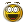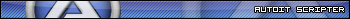Recommended Posts

I've find any where in in the internet and forum but could not find any solution for autoit function like encodeURIComponent  (javascript), you can see how function action by below link.

http://www.w3schools.com/jsref/jsref_encodeURIComponent.asp

My main point is to send a UTF8 data to one website, so that i have to encodeURIComponent  data to send it.

Share on other sites

I'm so exciting, now i can do that, autoit like PHP, that's one of my good languages, so that i found the PHP solution, then i convert it to my Autoit code, that's so good, the result is below.ConsoleWrite(encodeURIComponent("Tôi là người Việt Nam")&@CRLF)
ConsoleWrite(encodeURIComponent("我愛編碼這麼多，你愛我")&@CRLF)
ConsoleWrite(encodeURIComponent("я люблю кодирования так, ты меня любишь")&@CRLF)
Func encodeURIComponent(\$str)
Local \$i, \$return, \$tmp, \$exp
\$return = ""
\$exp = "[a-zA-Z0-9-._~]"
For \$i = 1 To StringLen(\$str)
\$tmp = StringMid(\$str, \$i, 1)
If StringRegExp(\$tmp, \$exp, 0) = 1 Then
\$return &= \$tmp
Else
\$return &= encodeURIComponentbycharacter(StringMid(StringRegExpReplace(StringToBinary(\$tmp, 4), "([0-9A-Fa-f]{2})", "%\$1"), 3))
EndIf
Next
Return \$return
EndFunc

Func encodeURIComponentbycharacter(\$char)
if (\$char = " ") Then return "%20"
if (\$char = "%21") Then return "!"
if (\$char = "%27") Then return '"'
if (\$char = "%28") Then return "("
if (\$char = "%29") Then return ")"
if (\$char = "%2A") Then return "*"
if (\$char = "%7E") Then return "~"
if (\$char = "%80") Then return "%E2%82%AC"
if (\$char = "%81") Then return "%C2%81"
if (\$char = "%82") Then return "%E2%80%9A"
if (\$char = "%83") Then return "%C6%92"
if (\$char = "%84") Then return "%E2%80%9E"
if (\$char = "%85") Then return "%E2%80%A6"
if (\$char = "%86") Then return "%E2%80%A0"
if (\$char = "%87") Then return "%E2%80%A1"
if (\$char = "%88") Then return "%CB%86"
if (\$char = "%89") Then return "%E2%80%B0"
if (\$char = "%8A") Then return "%C5%A0"
if (\$char = "%8B") Then return "%E2%80%B9"
if (\$char = "%8C") Then return "%C5%92"
if (\$char = "%8D") Then return "%C2%8D"
if (\$char = "%8E") Then return "%C5%BD"
if (\$char = "%8F") Then return "%C2%8F"
if (\$char = "%90") Then return "%C2%90"
if (\$char = "%91") Then return "%E2%80%98"
if (\$char = "%92") Then return "%E2%80%99"
if (\$char = "%93") Then return "%E2%80%9C"
if (\$char = "%94") Then return "%E2%80%9D"
if (\$char = "%95") Then return "%E2%80%A2"
if (\$char = "%96") Then return "%E2%80%93"
if (\$char = "%97") Then return "%E2%80%94"
if (\$char = "%98") Then return "%CB%9C"
if (\$char = "%99") Then return "%E2%84%A2"
if (\$char = "%9A") Then return "%C5%A1"
if (\$char = "%9B") Then return "%E2%80%BA"
if (\$char = "%9C") Then return "%C5%93"
if (\$char = "%9D") Then return "%C2%9D"
if (\$char = "%9E") Then return "%C5%BE"
if (\$char = "%9F") Then return "%C5%B8"
if (\$char = "%A0") Then return "%C2%A0"
if (\$char = "%A1") Then return "%C2%A1"
if (\$char = "%A2") Then return "%C2%A2"
if (\$char = "%A3") Then return "%C2%A3"
if (\$char = "%A4") Then return "%C2%A4"
if (\$char = "%A5") Then return "%C2%A5"
if (\$char = "%A6") Then return "%C2%A6"
if (\$char = "%A7") Then return "%C2%A7"
if (\$char = "%A8") Then return "%C2%A8"
if (\$char = "%A9") Then return "%C2%A9"
if (\$char = "%AA") Then return "%C2%AA"
if (\$char = "%AB") Then return "%C2%AB"
if (\$char = "%AC") Then return "%C2%AC"
if (\$char = "%AD") Then return "%C2%AD"
if (\$char = "%AE") Then return "%C2%AE"
if (\$char = "%AF") Then return "%C2%AF"
if (\$char = "%B0") Then return "%C2%B0"
if (\$char = "%B1") Then return "%C2%B1"
if (\$char = "%B2") Then return "%C2%B2"
if (\$char = "%B3") Then return "%C2%B3"
if (\$char = "%B4") Then return "%C2%B4"
if (\$char = "%B5") Then return "%C2%B5"
if (\$char = "%B6") Then return "%C2%B6"
if (\$char = "%B7") Then return "%C2%B7"
if (\$char = "%B8") Then return "%C2%B8"
if (\$char = "%B9") Then return "%C2%B9"
if (\$char = "%BA") Then return "%C2%BA"
if (\$char = "%BB") Then return "%C2%BB"
if (\$char = "%BC") Then return "%C2%BC"
if (\$char = "%BD") Then return "%C2%BD"
if (\$char = "%BE") Then return "%C2%BE"
if (\$char = "%BF") Then return "%C2%BF"
if (\$char = "%C0") Then return "%C3%80"
if (\$char = "%C1") Then return "%C3%81"
if (\$char = "%C2") Then return "%C3%82"
if (\$char = "%C3") Then return "%C3%83"
if (\$char = "%C4") Then return "%C3%84"
if (\$char = "%C5") Then return "%C3%85"
if (\$char = "%C6") Then return "%C3%86"
if (\$char = "%C7") Then return "%C3%87"
if (\$char = "%C8") Then return "%C3%88"
if (\$char = "%C9") Then return "%C3%89"
if (\$char = "%CA") Then return "%C3%8A"
if (\$char = "%CB") Then return "%C3%8B"
if (\$char = "%CC") Then return "%C3%8C"
if (\$char = "%CD") Then return "%C3%8D"
if (\$char = "%CE") Then return "%C3%8E"
if (\$char = "%CF") Then return "%C3%8F"
if (\$char = "%D0") Then return "%C3%90"
if (\$char = "%D1") Then return "%C3%91"
if (\$char = "%D2") Then return "%C3%92"
if (\$char = "%D3") Then return "%C3%93"
if (\$char = "%D4") Then return "%C3%94"
if (\$char = "%D5") Then return "%C3%95"
if (\$char = "%D6") Then return "%C3%96"
if (\$char = "%D7") Then return "%C3%97"
if (\$char = "%D8") Then return "%C3%98"
if (\$char = "%D9") Then return "%C3%99"
if (\$char = "%DA") Then return "%C3%9A"
if (\$char = "%DB") Then return "%C3%9B"
if (\$char = "%DC") Then return "%C3%9C"
if (\$char = "%DD") Then return "%C3%9D"
if (\$char = "%DE") Then return "%C3%9E"
if (\$char = "%DF") Then return "%C3%9F"
if (\$char = "%E0") Then return "%C3%A0"
if (\$char = "%E1") Then return "%C3%A1"
if (\$char = "%E2") Then return "%C3%A2"
if (\$char = "%E3") Then return "%C3%A3"
if (\$char = "%E4") Then return "%C3%A4"
if (\$char = "%E5") Then return "%C3%A5"
if (\$char = "%E6") Then return "%C3%A6"
if (\$char = "%E7") Then return "%C3%A7"
if (\$char = "%E8") Then return "%C3%A8"
if (\$char = "%E9") Then return "%C3%A9"
if (\$char = "%EA") Then return "%C3%AA"
if (\$char = "%EB") Then return "%C3%AB"
if (\$char = "%EC") Then return "%C3%AC"
if (\$char = "%ED") Then return "%C3%AD"
if (\$char = "%EE") Then return "%C3%AE"
if (\$char = "%EF") Then return "%C3%AF"
if (\$char = "%F0") Then return "%C3%B0"
if (\$char = "%F1") Then return "%C3%B1"
if (\$char = "%F2") Then return "%C3%B2"
if (\$char = "%F3") Then return "%C3%B3"
if (\$char = "%F4") Then return "%C3%B4"
if (\$char = "%F5") Then return "%C3%B5"
if (\$char = "%F6") Then return "%C3%B6"
if (\$char = "%F7") Then return "%C3%B7"
if (\$char = "%F8") Then return "%C3%B8"
if (\$char = "%F9") Then return "%C3%B9"
if (\$char = "%FA") Then return "%C3%BA"
if (\$char = "%FB") Then return "%C3%BB"
if (\$char = "%FC") Then return "%C3%BC"
if (\$char = "%FD") Then return "%C3%BD"
if (\$char = "%FE") Then return "%C3%BE"
if (\$char = "%FF") Then return "%C3%BF"
return \$char
EndFunc

Edited by drquochoai

Share on other sites

Did you try a forum search.

\$url= "http://w3schools.com/my test.asp?name=ståle&car=saab";
ConsoleWrite(\$url & @crlf)
ConsoleWrite(_URIEncode(\$url) & @CRLF)

Func _URIEncode(\$sData)
; Prog@ndy
Local \$aData = StringSplit(BinaryToString(StringToBinary(\$sData,4),1),"")
Local \$nChar
\$sData=""
For \$i = 1 To \$aData
; ConsoleWrite(\$aData[\$i] & @CRLF)
Switch \$nChar
Case 45, 46, 48 To 57, 65 To 90, 95, 97 To 122, 126
;~             Case 32
;~                 \$sData &= "+"
Case Else
\$sData &= "%" & Hex(\$nChar,2)
EndSwitch
Next
Return \$sData
EndFunc

SaludosDanysys.comAutoIt...

Share on other sites

wao, that's so good, did not find that function before, thanks Danyfirex so much.

Create an account or sign in to comment

You need to be a member in order to leave a comment

Create an account

Sign up for a new account in our community. It's easy!

Register a new account